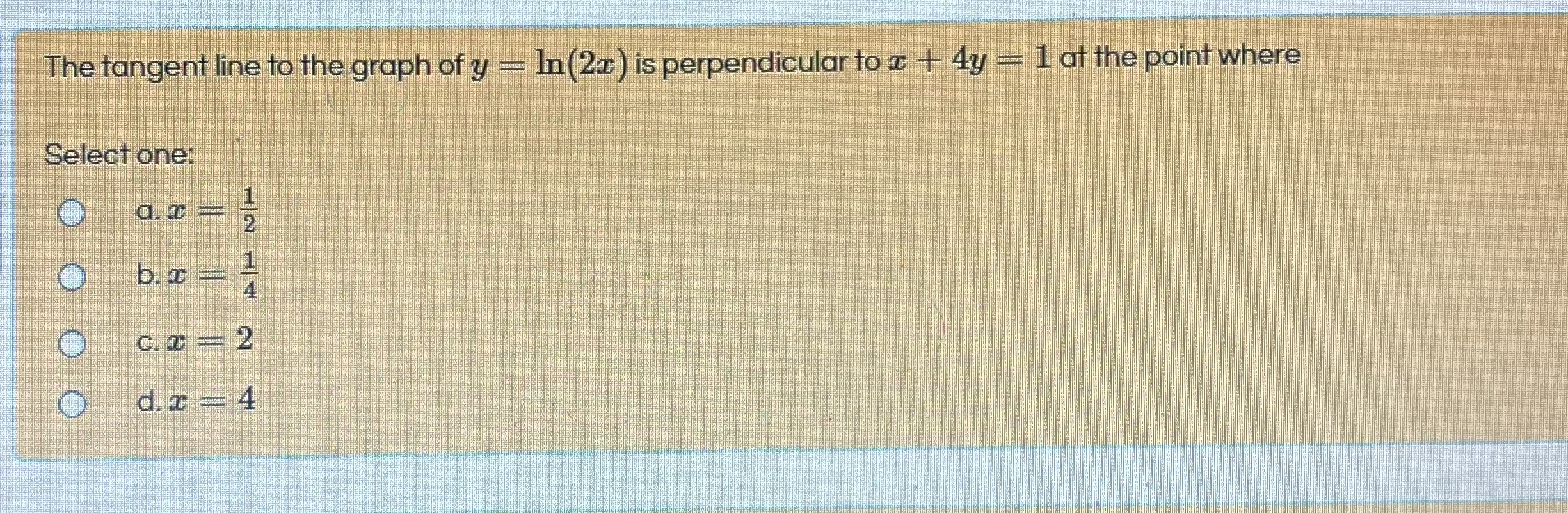# The tangent line to the graph of y = In(2x) is perpendicular to a + 4y = 1 at the point where Select one: a. 2 b. ¤ = C. = 2 d. z = 4 1/21/4

Questionhelp_outlineImage TranscriptioncloseThe tangent line to the graph of y = In(2x) is perpendicular to a + 4y = 1 at the point where Select one: a. 2 b. ¤ = C. = 2 d. z = 4 1/21/4 fullscreen

### Want to see this answer and more?

Experts are waiting 24/7 to provide step-by-step solutions in as fast as 30 minutes!*

*Response times vary by subject and question complexity. Median response time is 34 minutes and may be longer for new subjects.
Tagged in
MathCalculus

### Applications of Derivative